Next: Results Up: Linear analysis of Previous: Long-range clustered inhibitory

## The Resultant Receptive Fields

From (10), the distribution of excitation on the cortical plane can be explicitly rewritten as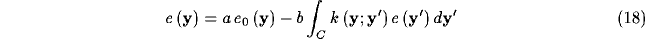where, see equations (9) and (11)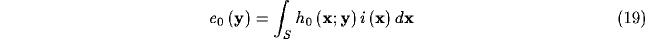According to the method of Fredholm for inhomogeneous integral equations  an approximate solution of equation (18) can be expressed in the form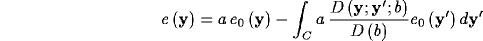where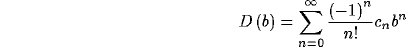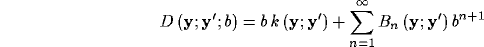Substituting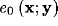from (19) and comparing the result with (8) we identify the kernel and thence the resulting RF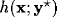after recurrent inhibition: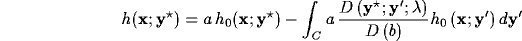Making explicit the parametric mapping between cortical plane (C) and visual field (S), the resulting RF can be formulated as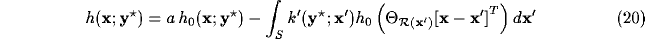where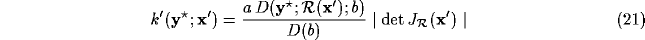is the equivalent inhibitory kernel in the feed-forward form.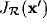denotes the Jacobian of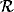and the absolute value of its determinant is the local magnification factor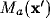of the mapping. A more detailed derivation of this solution can be found in the Appendix.Next: Results Up: Linear analysis of Previous: Long-range clustered inhibitory